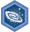## FANDOM

1,895 Pages

8th grade (U.S.): Linear equations and functionsDescription
Achieve mastery in all skills in 8th grade (U.S.): Linear equations and functions
Details
TypeEnergy point(s) awarded 0
Status Available
Disclosed?
Repeating?

This page contains exercises which pertain to the subcategory 8th grade (U.S.): Linear equations and functions. Mastering them awards user with the Challenge Patch 8th grade (U.S.): Linear equations and functions as well.

All items (31)

C
•Checking solutions to two-variable linear equations
•Comparing linear functions
•Comparing linear functions word problems
•Complete missing values in linear equations according to the number of solutions
•Constructing linear functions word problems
E
•Evaluating functions
G
•Generating input-output pairs of a function
•Graph from slope-intercept equation
•Graphing linear functions word problems
•Graphing proportional relationships
•Graphing solutions to two-variable linear equations
I
•Intercepts from a graph
•Intercepts from a linear equation
•Interpreting graphs of linear and nonlinear functions
•Interpreting linear formulas word problems
•Interpreting linear graphs word problems
L
•Linear and nonlinear functions
•Linear models word problems
R
•Rates and proportional relationships
•Recognizing functions from graphs
•Recognizing functions from tables
S
•Slope from a graph
•Slope from an equation in slope-intercept form
•Slope from an equation in standard form
•Slope from two solutions
•Slope intuition
•Slope-intercept equation from a graph
•Slope-intercept equation from two solutions
•Solve systems of linear equations with elimination (advanced)
•Solve systems of linear equations with substitution
W
•Writing function rules from equations